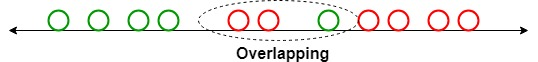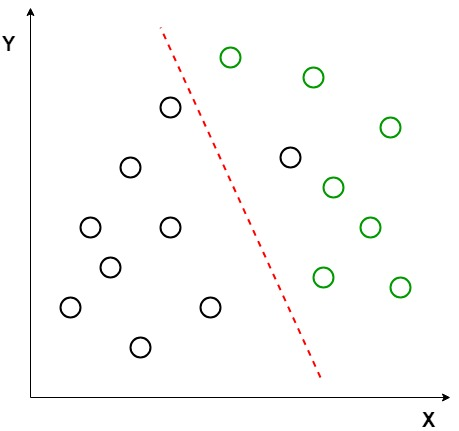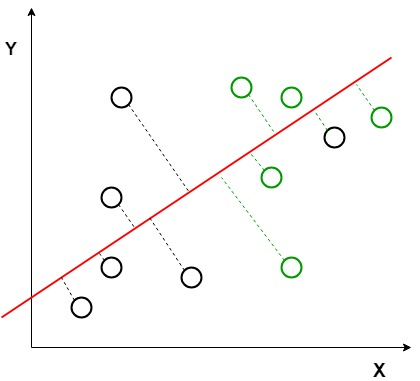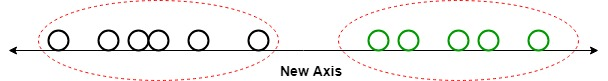# ML | Linear Discriminant Analysis

Linear Discriminant Analysis or Normal Discriminant Analysis or Discriminant Function Analysis is a dimensionality reduction technique which is commonly used for the supervised classification problems. It is used for modeling differences in groups i.e. separating two or more classes. It is used to project the features in higher dimension space into a lower dimension space.

For example, we have two classes and we need to separate them efficiently. Classes can have multiple features. Using only a single feature to classify them may result in some overlapping as shown in the below figure. So, we will keep on increasing the number of features for proper classification.Example:
Suppose we have two sets of data points belonging to two different classes that we want to classify. As shown in the given 2D graph, when the data points are plotted on the 2D plane, there’s no straight line that can separate the two classes of the data points completely. Hence, in this case, LDA (Linear Discriminant Analysis) is used which reduces the 2D graph into a 1D graph in order to maximize the separability between the two classes.Here, Linear Discriminant Analysis uses both the axes (X and Y) to create a new axis and projects data onto a new axis in a way to maximize the separation of the two categories and hence, reducing the 2D graph into a 1D graph.

Two criteria are used by LDA to create a new axis:

1. Maximize the distance between means of the two classes.
2. Minimize the variation within each class.In the above graph, it can be seen that a new axis (in red) is generated and plotted in the 2D graph such that it maximizes the distance between the means of the two classes and minimizes the variation within each class. In simple terms, this newly generated axis increases the separation between the dtla points of the two classes. After generating this new axis using the above-mentioned criteria, all the data points of the classes are plotted on this new axis and are shown in the figure given below.But Linear Discriminant Analysis fails when the mean of the distributions are shared, as it becomes impossible for LDA to find a new axis that makes both the classes linearly separable. In such cases, we use non-linear discriminant analysis.

Extensions to LDA:

1. Quadratic Discriminant Analysis (QDA): Each class uses its own estimate of variance (or covariance when there are multiple input variables).
2. Flexible Discriminant Analysis (FDA): Where non-linear combinations of inputs is used such as splines.
3. Regularized Discriminant Analysis (RDA): Introduces regularization into the estimate of the variance (actually covariance), moderating the influence of different variables on LDA.

Applications:

1. Face Recognition: In the field of Computer Vision, face recognition is a very popular application in which each face is represented by a very large number of pixel values. Linear discriminant analysis (LDA) is used here to reduce the number of features to a more manageable number before the process of classification. Each of the new dimensions generated is a linear combination of pixel values, which form a template. The linear combinations obtained using Fisher’s linear discriminant are called Fisher faces.
2. Medical: In this field, Linear discriminant analysis (LDA) is used to classify the patient disease state as mild, moderate or severe based upon the patient various parameters and the medical treatment he is going through. This helps the doctors to intensify or reduce the pace of their treatment.
3. Customer Identification: Suppose we want to identify the type of customers which are most likely to buy a particular product in a shopping mall. By doing a simple question and answers survey, we can gather all the features of the customers. Here, Linear discriminant analysis will help us to identify and select the features which can describe the characteristics of the group of customers that are most likely to buy that particular product in the shopping mall.
My Personal Notes arrow_drop_upCheck out this Author's contributed articles.

If you like GeeksforGeeks and would like to contribute, you can also write an article using contribute.geeksforgeeks.org or mail your article to contribute@geeksforgeeks.org. See your article appearing on the GeeksforGeeks main page and help other Geeks.

Please Improve this article if you find anything incorrect by clicking on the "Improve Article" button below.

Article Tags :
Practice Tags :

1

Please write to us at contribute@geeksforgeeks.org to report any issue with the above content.